Part 20: On the structure of non-invertible 4D complex numbers.

In general it is rather hard to find non-invertible 4D complex numbers because the determinant is non-negative everywhere. Just try it yourself, write down just one 4D complex number that is non-zero and not invertible.

That is not an easy task, after some time you will find some but do you have all?

But if you understand the concept of the eigenvalues that every 4D complex number Z has, it is easy to understand that if a 4D complex number is non-invertible at least one of the eigenvalues must be zero.

In previous posts we already unearthed the four eigenvalue functions that return the four eigenvalues each 4D complex number has.

In this post we will try to find where these eigenvalue functions are zero.

Since eigenvalue functions come in pairs whenever possible in the case of 4D complex numbers we only have two pairs of those eigenvalue functions.

Let’s stop the talking and just post the twelve pictures that make up part number 20 into the basics of the four dimensional complex numbers.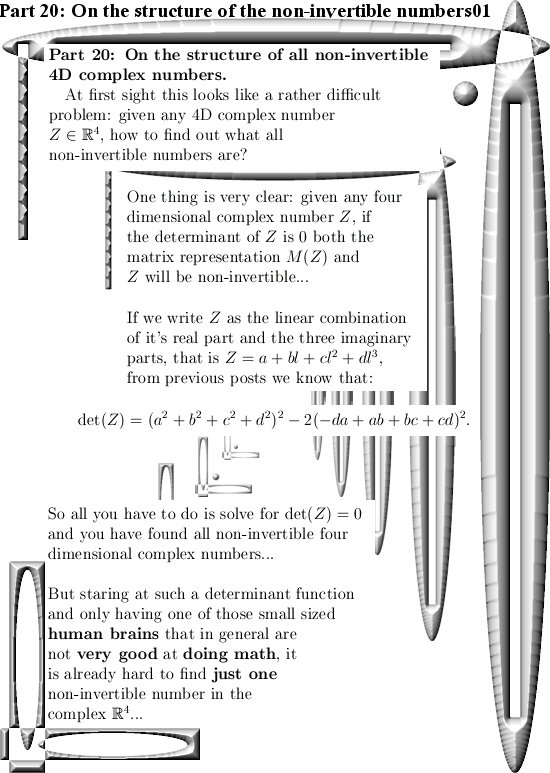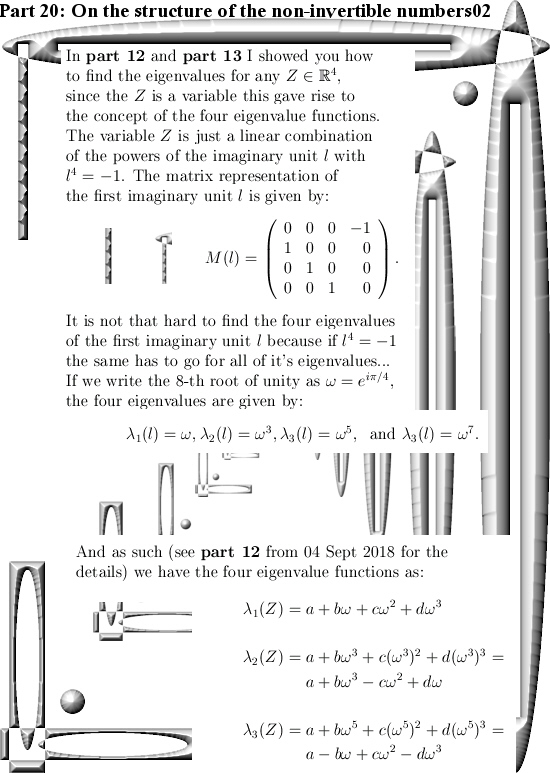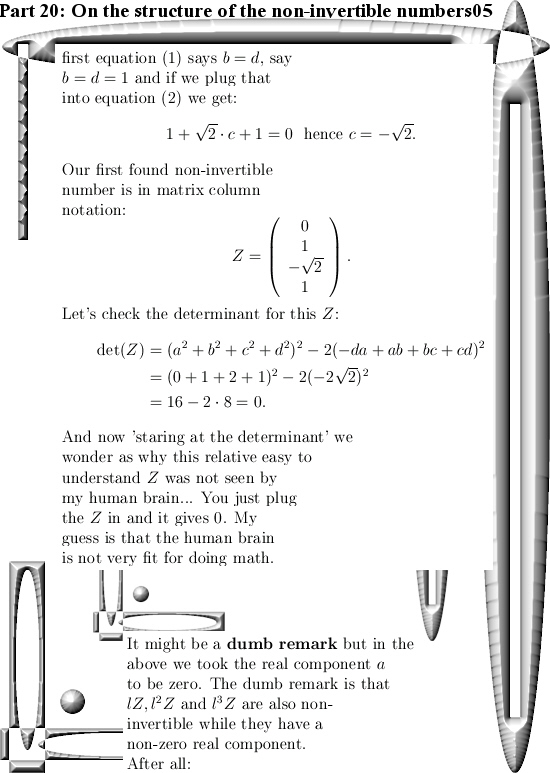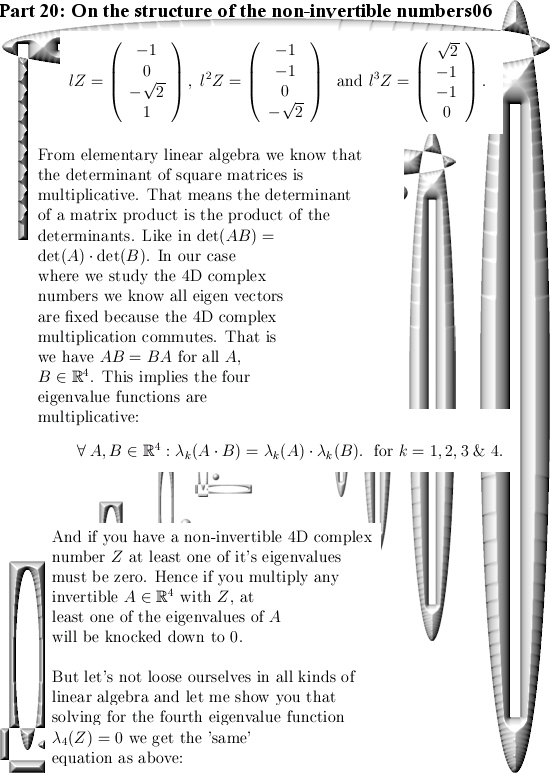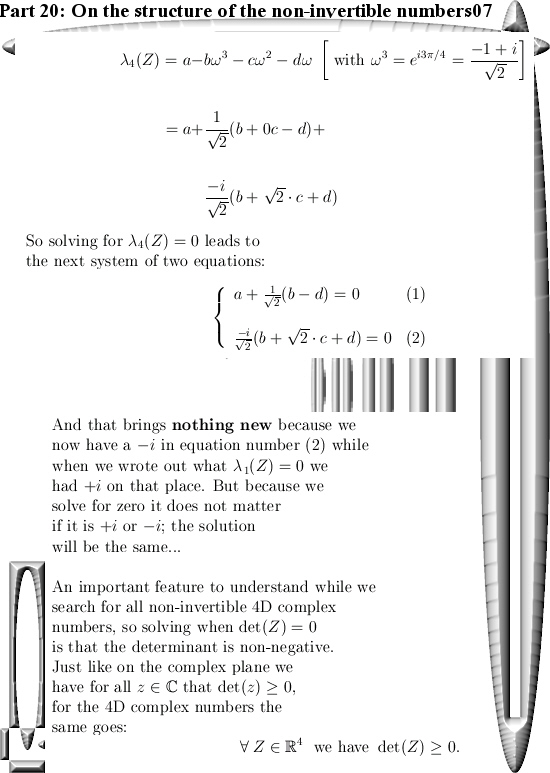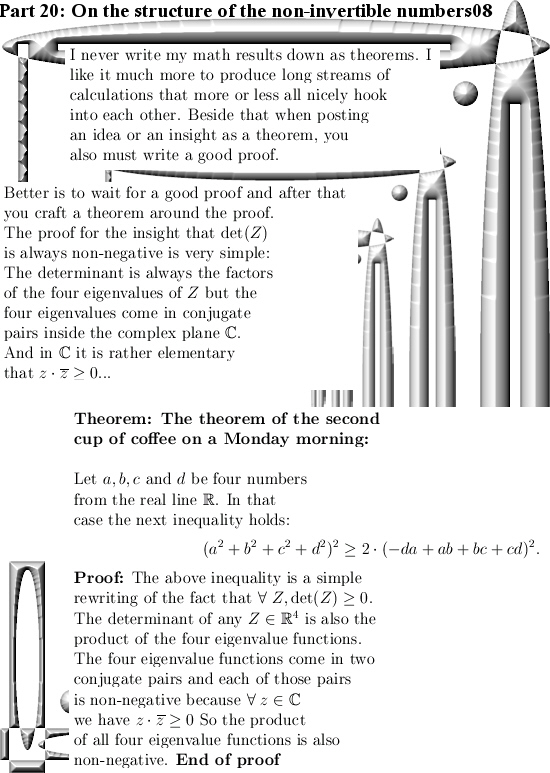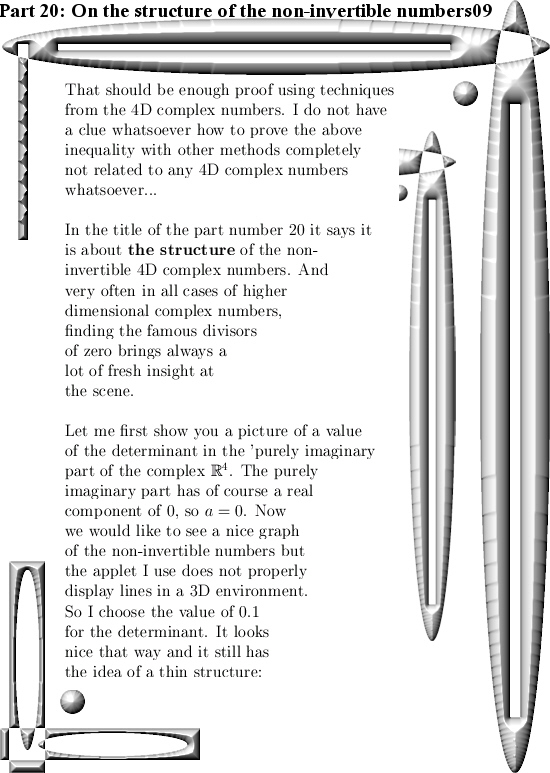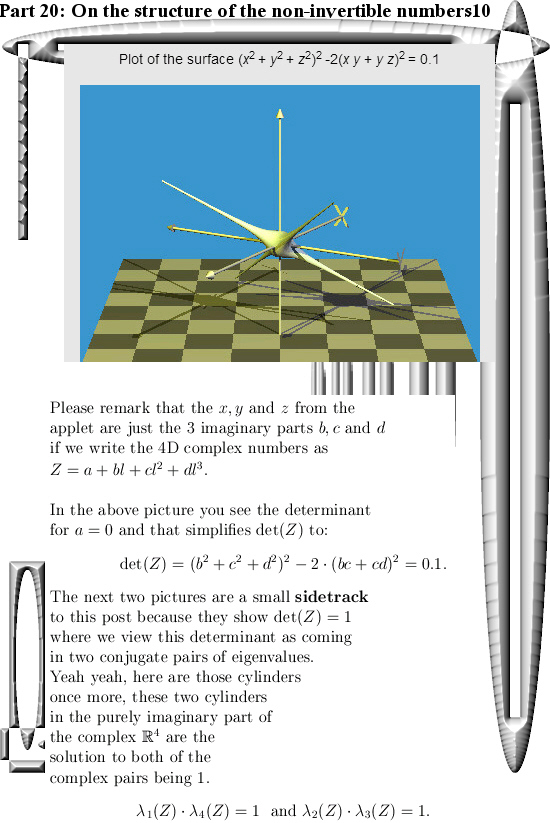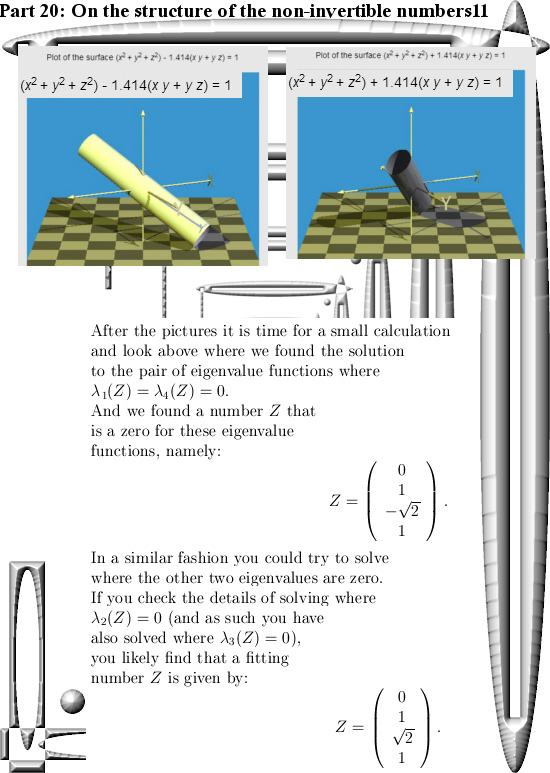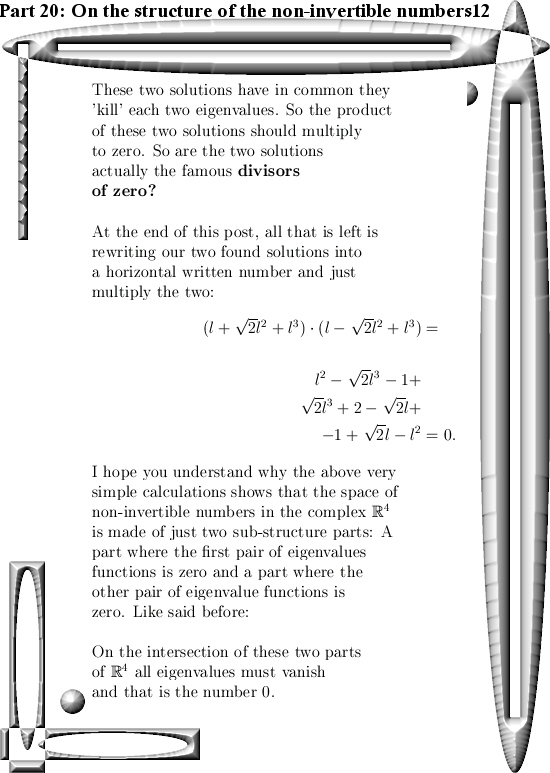Ok, that was it for part 20 in the series that covers the basics of 4D complex numbers.

See you around my dear reader.

A teaser question: Can you prove this inequality?

Recently I am working on part 20 to the basics of the 4D complex numbers. Ok ok if you need 20 parts to explain ‘the basics’ how basic is it you can ask yourself.

You can argue long and short on this: are fresh Cauchy integral formula’s really ‘basic stuff’? I don’t know how a democratic vote among professional math professors would fall down.

Anyway, an important property of the determinant of 4D complex numbers is the fact that the determinant is always non-negavite. At least it is zero and at those points in space we have found a non-invertible number.

In part 20 on the basics to 4D complex numbers we will look when the eigenvalues of 4D complex numbers vanish; at those points the stuff is non-invertible & that is what we will be hunting on part 20.

In the next picture you see a difficult to understand inequality & the teaser question is:
Can you prove this inequality via math methods that do not use 4D complex number theory at all?

If so, you should definitely pop up a second pint of perfect beer on a late Friday evening.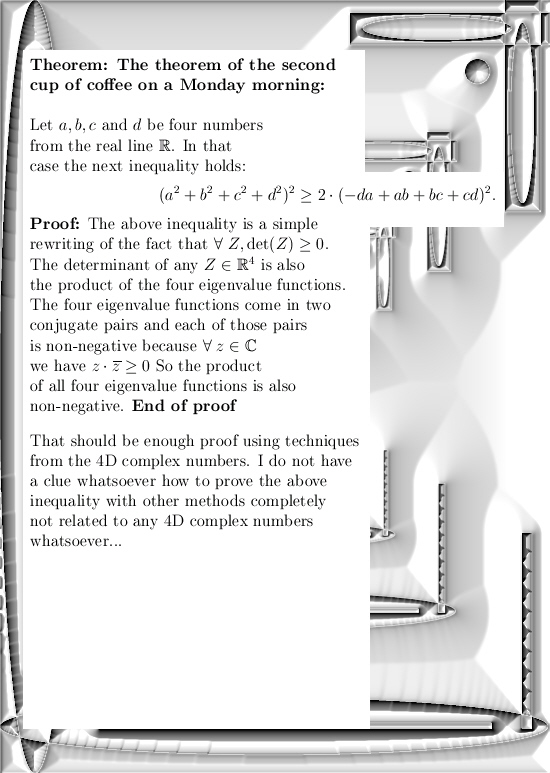Ok, that was it. Till updates in part 20 where we try to find all non-invertible 4D complex numbers in a not too difficult way.

Part 19: Four integrals defining the 4D complex number tau.

It is a bit late but a happy new year anyway! In this post we will do a classic from the complex plane: calculation of the log of the first imaginary unit.

On the complex plane this is log i and on the complex 4D space this is log l .

Because this number is so important I have given it a separate name a long long time ago: These are the numbers tau in the diverse dimensions. In the complex plane it has no special name and it simply is i times pi/2.

On the real line it is pretty standard to define the log functions as the integral of the inverse 1/x. After all the derivative of log x on the real line is 1/x and as such you simply define the log to be the integral of the derivative…

On the complex plane you can do the same but depending of how your path goes around zero you can get different answers. Also in the complex plane (and other higher dimensional number systems) the log is ‘multi valued’. That is a reflection of the fact we can find exponential periodic functions also known as the exponential circles and curves.

The integrals in this part number 19 on the basics of 4D complex numbers are very hard to crack. I know of no way to find primitives and to crack them that way. May be that is possible, may be it is not, I just do not know. But because I developed the method of matrix diagonals for finding expressions for the value of those difficult looking integrals, more or less in an implicit manner we give the right valuations to those four integrals.

With the word ‘implicit’ I simply mean we skip the whole thing of caculating the number tau via matrix diagonalization. We only calculate what those integrals actually are in terms of a half circle with coordinates cos t and sin t.

This post is 8 pictures long in the usual size of 550 by 775 pixels (I had to enlarge the latest picture a little bit). I hope it is not loaded with typo’s any more and you have a more or less clean mathematical experience: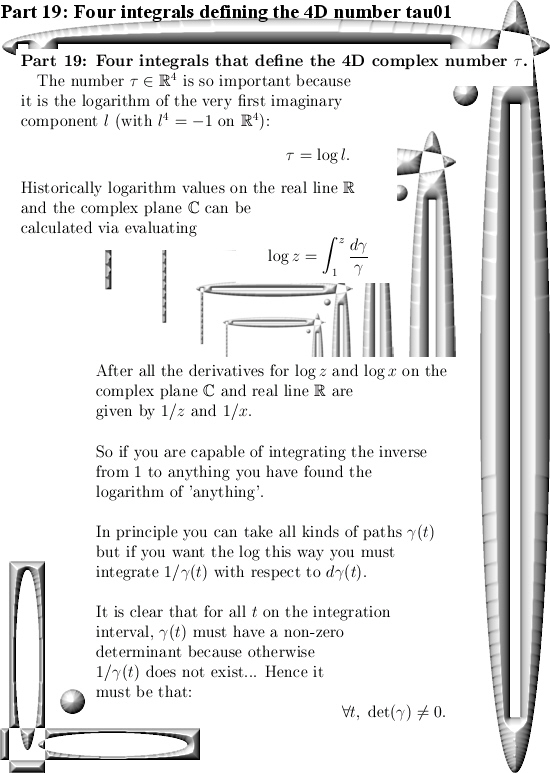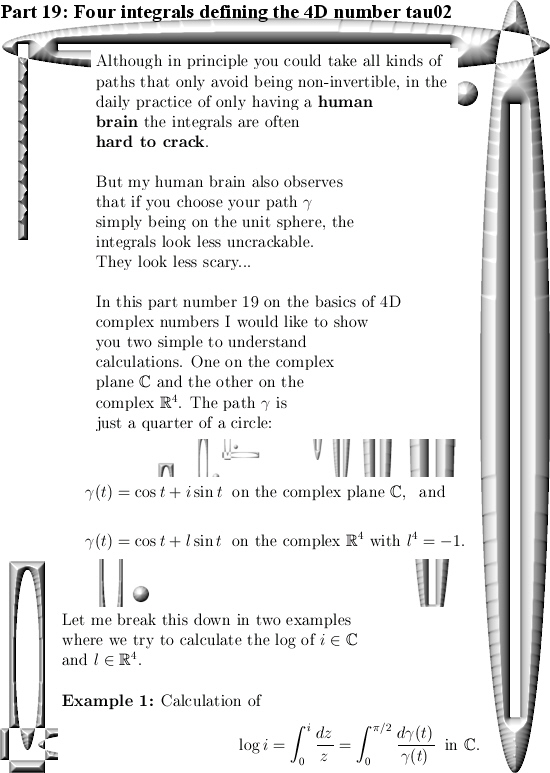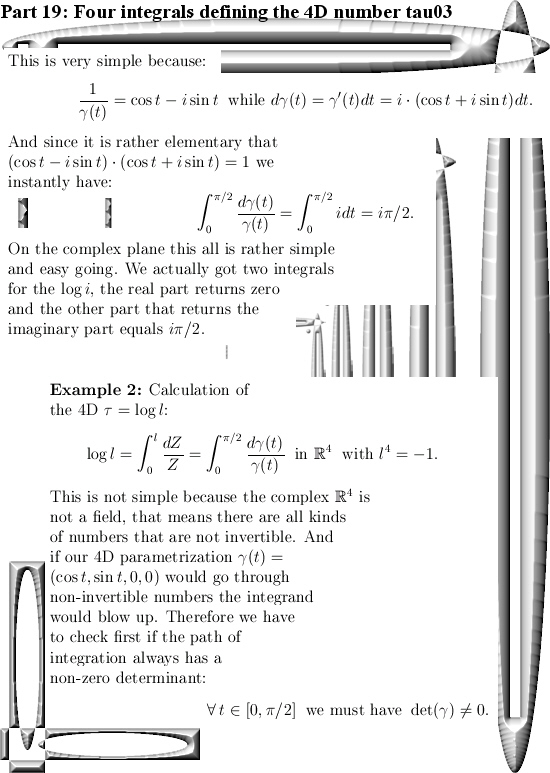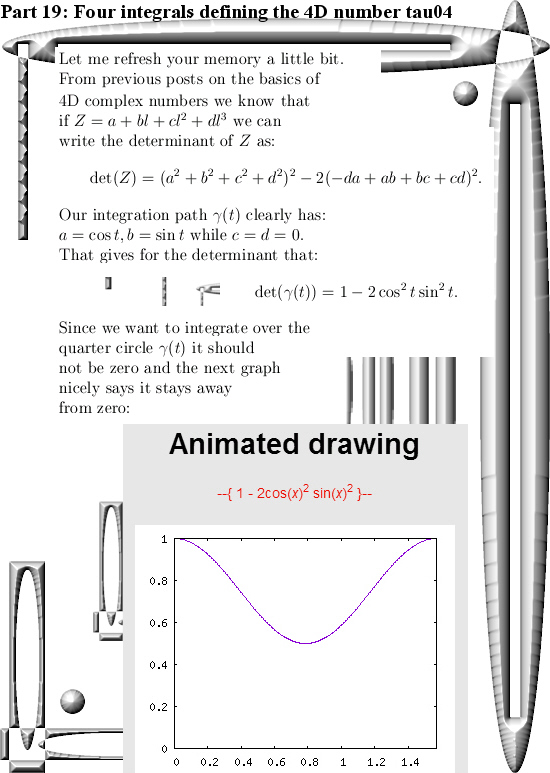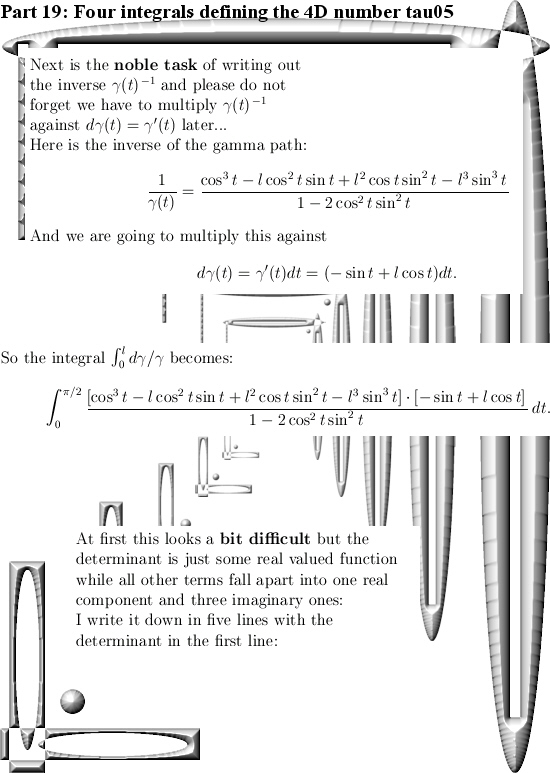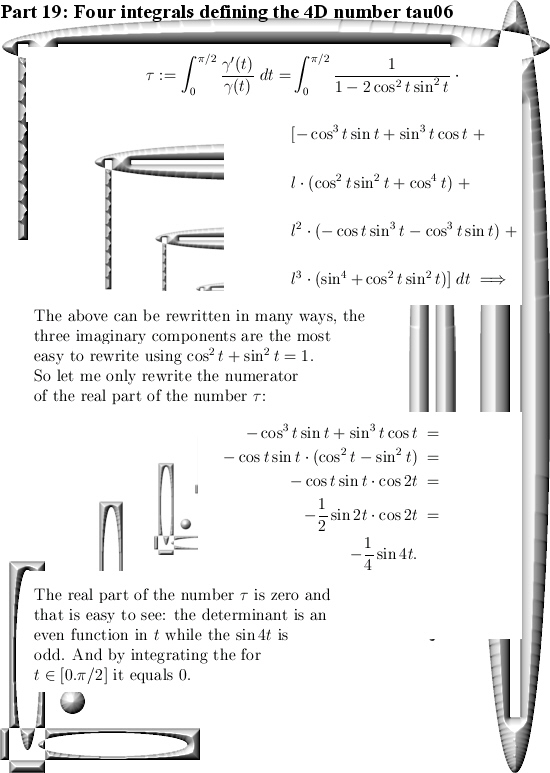End of this post.

Part 18: Calculating the 4D number tau it’s inverse in a very simple way.

It is the shortest day of the year today and weirdly enough I like this kind of wether better compared to the extreme heat of last summer. Normally I dislike those long dark days but after so much heat for so long I just don’t mind the darkness and the tiny amounts of cold.

In the previous post we found a general way of finding all inverses possible in the space of the 4D complex numbers. Furthermore in the post with the new Cauchy integral representation we had to make heavy use of 1/8tau and as such it is finally time to look at what the inverse of tau actually is.

I found a very simple way of calculating the inverse of the number tau. It boils down to solving a system of two linear equations in two variables. As far as I know reality, most math professionals can actually do this. Ok ok, for the calculation to be that simple you first must assume that the inverse ‘looks like’ the number tau in the sense it has no real component and it is just like tau a linear combination of those so called ‘imitators of i‘.

This is a short post, only five pictures long. I started the 4D complex number stuff somewhere in April of this year so it is only 8 month down the timeline that we look at the 4D complex numbers. It is interesting to compare the behavior of the average math professor to back in the time to Hamilton who found the 4D quaternions.

Hamilton became sir Hamilton rather soon (although I do not know why he became a noble man) and what do I get? Only silence year in year out. You see the difference between present day and past centuries is the highly inflated ego of the present day university professors. Being humble is not something they are good at…

After having said that, here are the five pictures: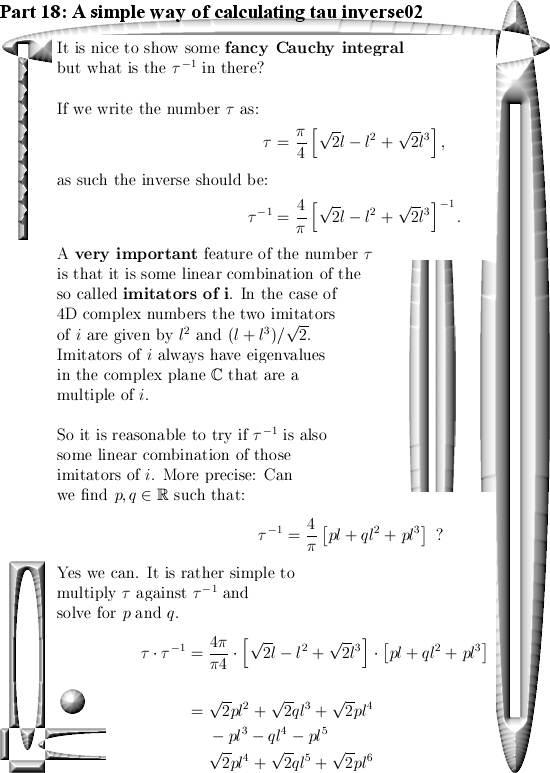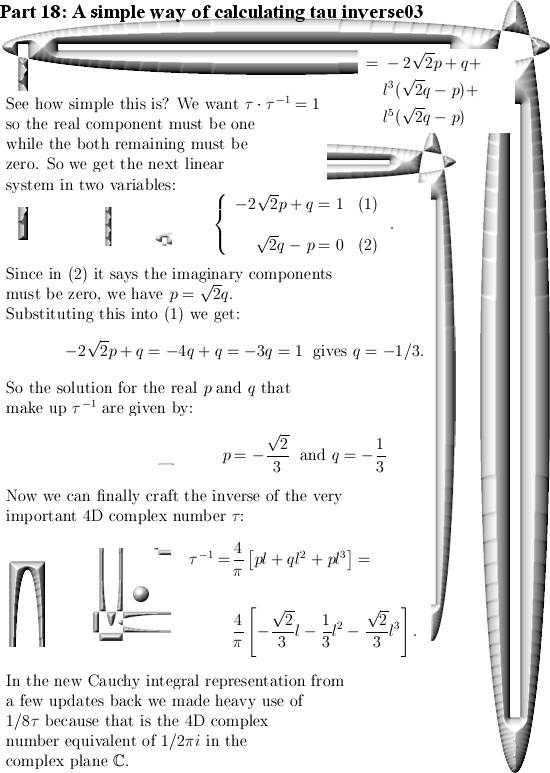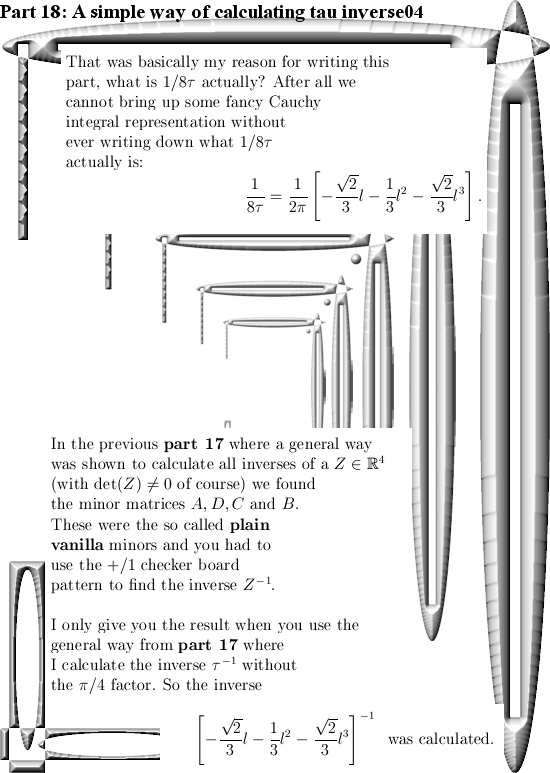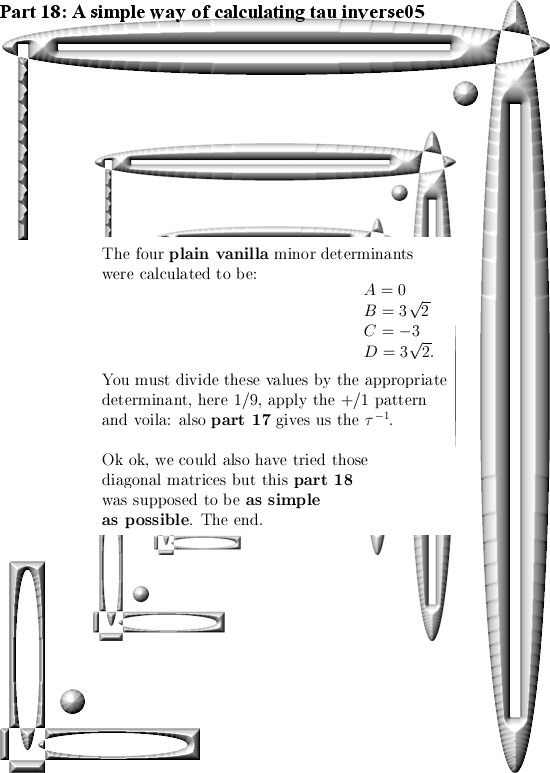All in all I have begun linking the 4D complex numbers more and more in the last 8 months. On details the 4D complex numbers are very different compared to 3D and say 5D complex numbers but there are always reasons for that. For example the number tau has an inverse in the space of 4D complex numbers but this is not the case in 3 or 5D complex numbers space.

Well, have a nice Christmas & likely see you in the next year 2019.

Part 17: The inverse of a 4D complex number old school style (via minor matrices).

Ha, a couple of weeks back I met an old colleague and it was nice to see him. We made a bit of small talk and more or less all of a sudden he said: ‘But you still can always do this’. And he meant getting a PhD in math.

I was a bit surprised he did bring this up, for me that was a station passed long ago. But he made me thinking a bit, why am I not interested in getting a math degree?

And when I thought it out I also had to laugh: Those people cannot go beyond the complex plane for let’s say 250 years. And the only people I know of that have studied complex numbers beyond the complex plane are all non-math people. Furthermore inside math there is that cultural thing that more or less says that if you try to find complex numbers beyond the complex plane, you must have a ‘mental thing’ because have you never heard of the 2-4-8 theorem?

Beside this, if I tried it in the years 1990 and 1991 with very simple: Here this is how the 3D Cauchy Riemann equations look… And you look them in the eyes, but there is nothing happening behind those eyes or in the brain of that particular math professor. Why the hell should I return and under the perfect guidance of such a person get a PhD?

I am not a masochist. If complex numbers beyond the complex plane are ignored, why try to change this? After all this is a free world and most societies run best when people can do what they are good at. Apparently math like I make simply falls off the radar screen, I do not have much problems with that.

___________

After having said that, this update Part 17 in the basics to the 4D complex numbers is as boring as possible. Just finding the inverse of a matrix just like in linear algebra with the method of minor matrices.

Believe me it is boring as hell. And after all that boring stuff only one small glimmer of light via crafting a very simple factorization of the determinant inside the 4D complex numbers. So that is very different from the previous factorization where we multiplied the four eigenvalue functions. From the math point it is a shallow result because it is so easy to find but when before your very own eyes you see the determinant arising from those calculations, it is just beautiful. And may be we should be striving a tiny bit more upon mathematical beauty…

This post is nine pictures long in the usual size of 550×775 pixels.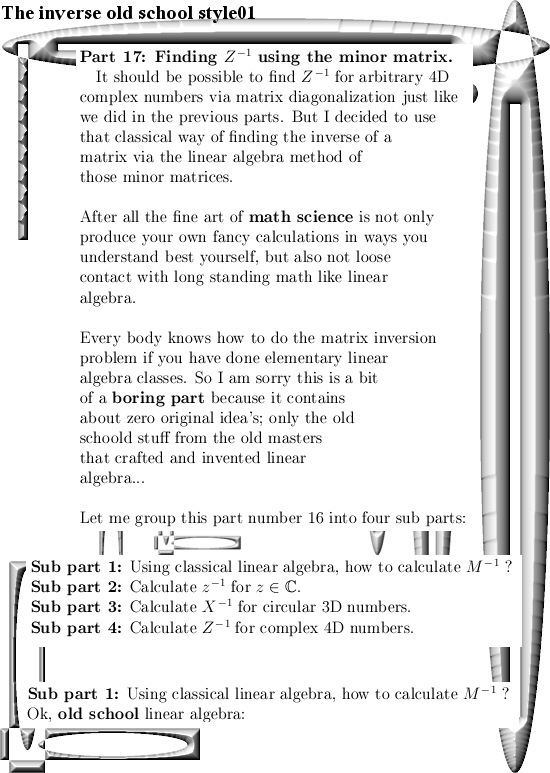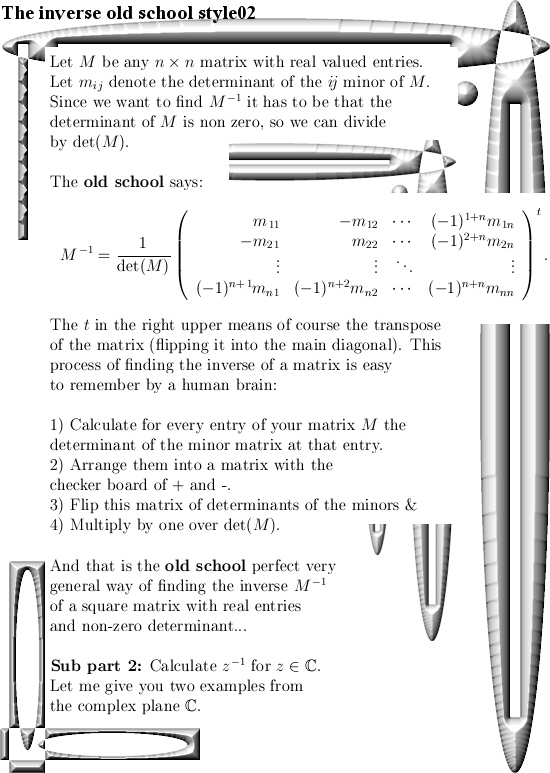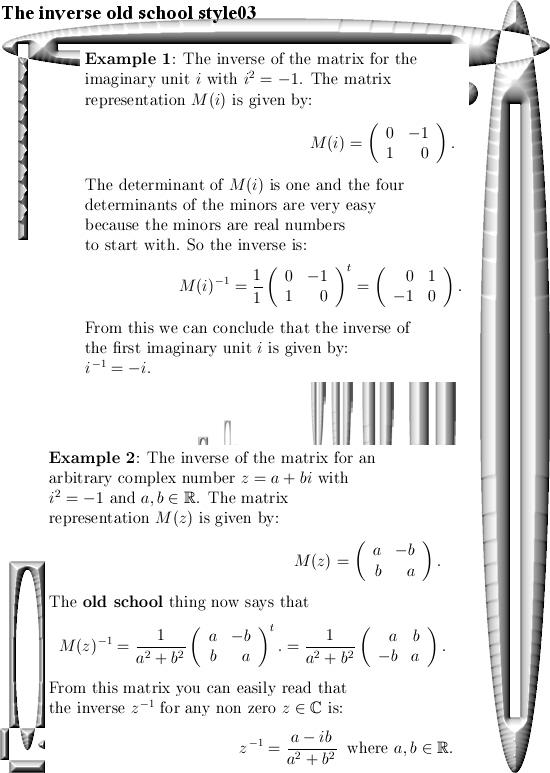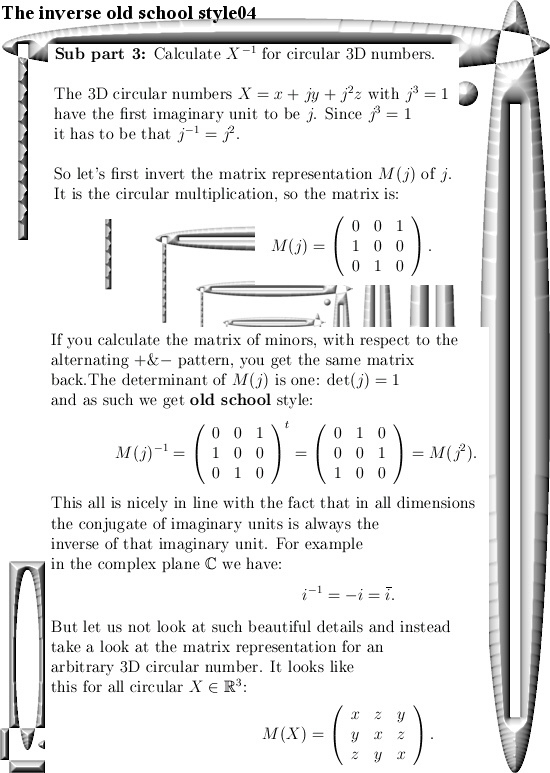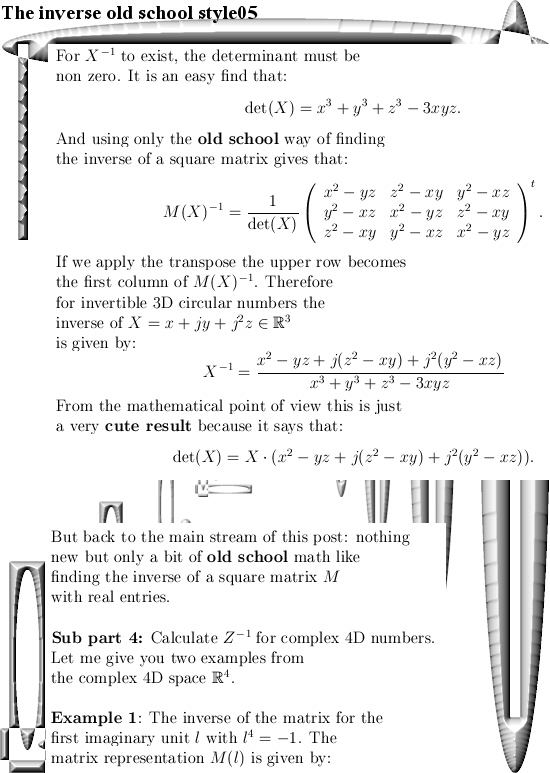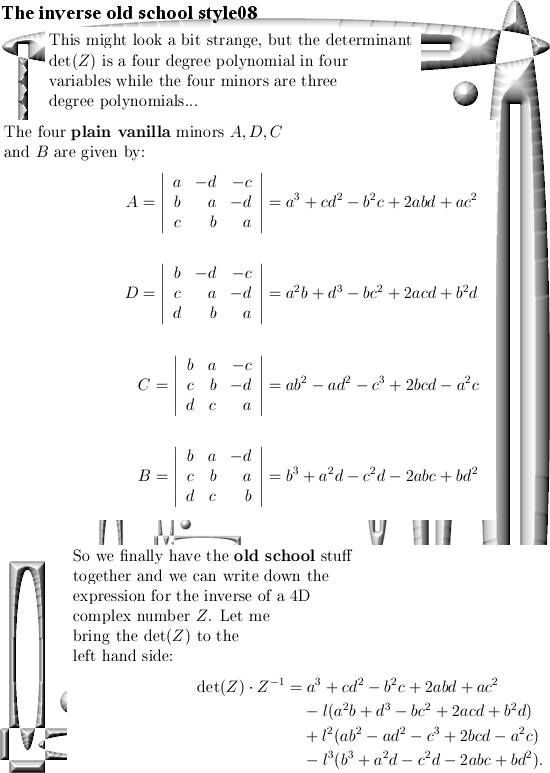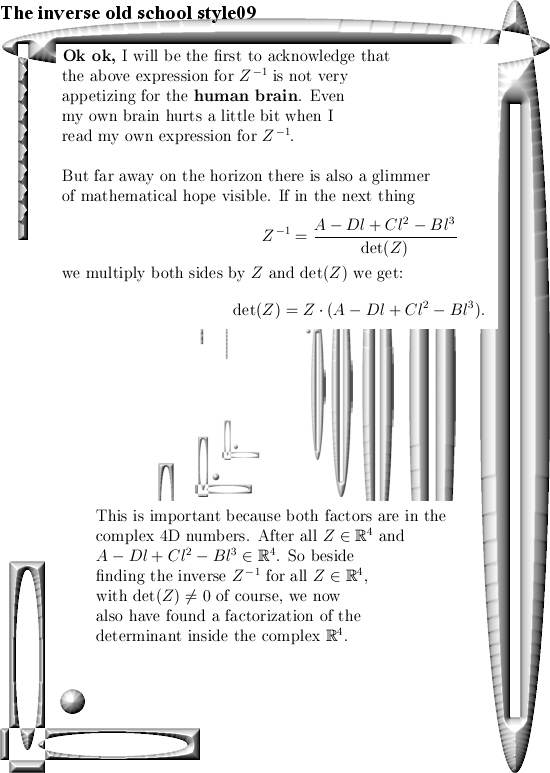As an antidote against so much polynomials like det(Z), with 2 dimensions like a flatscreen television, you can do a lot of fun too. The antidote is a video from the standupmaths guy, it is very funny and has the title ‘Infinite DVD unboxing video: Festival of the Spoken Nerd’. Here is the vid:

End of this update, see you around.

Ok ok, a few days later I decided to write a small appendix to this post and in order too keep it simple let’s calculate the determinant of the 3D circular numbers. I have to admit this is shallow math but despite being shallow it gives a crazy way to calculate the determinant of a 3D circular number…

So a small appendix, here it is: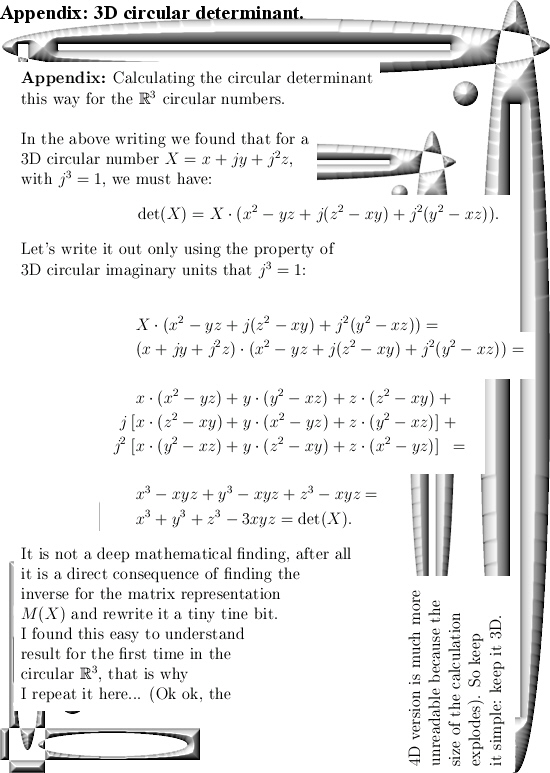And now you are really at the end of this post.

In the next post let’s calculate the inverse of the 4D complex tau number. After all a few months back I gave you the new Cauchy integral representation and I only showed that the determinant of tau was nonzero.

But the fact that the Cauchy integral representation is so easy to craft on the 4D complex numbers arises from the fact the inverse of tau exists in the first place. In 3D the number tau is not invertible, and Cauchy integral representations are much more harder to find.

Ok, drink a green tea or pop up a fresh pint, till updates.

Calculation of the 4D number tau diagonal matrix style.

In the begin of this series on basic and elementary calculations you can do with 4D complex numbers we already found what the number tau is. We used stuff like the pull back map… But you can do it also with the method from the previous post about how to find the matrix representation for any 4D complex number Z given the eigenvalues.

Finding the correct eigenvalues for tau is rather subtle, you must respect the behavior of the logarithm function in higher dimensions. It is not as easy as on the real line where you simply have log ab = log a + log b for positive reals a and b.

But let me keep this post short and stop all the blah blah.

Just two nice pictures is all to do the calculation of the 4D complex number tau: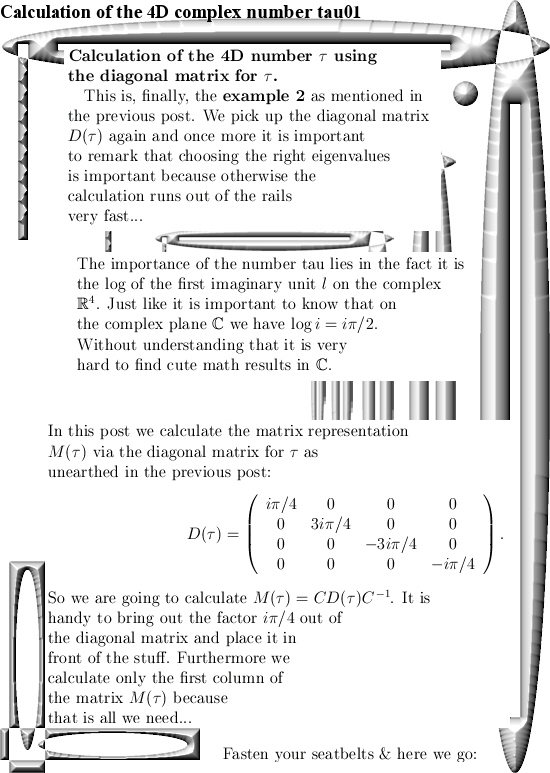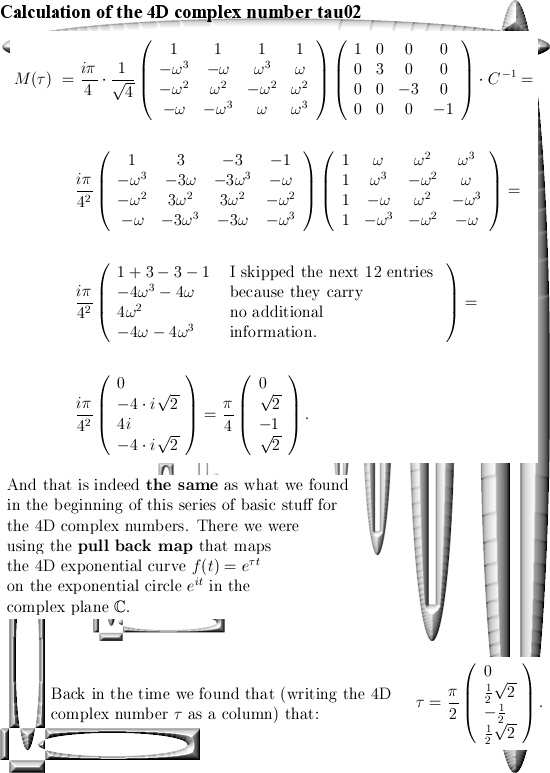(Oops, two days later I repaired a silly typo where I did forget one minus sign. It was just a dumb typo that likely did not lead to much confusion. So I will not take it in the ‘Corrections’ categorie on this website that I use for more or less more significant repairs…)

Ok, that was it.

Diagonal matrices for all 4D complex numbers.

This website is now about 3 years old, the first post was on 14 Nov 2015 and today I hang in with post number 100. That is a nice round number and this post is part 15 in the series known as the Basics for 4D complex numbers.

We are going to diagonalize all those matrix representations M(Z) we have for all 4D complex numbers Z. As a reader you are supposed to know what diagonalization of a matrix actually is, that is in most linear algebra courses so it is widely spread knowledge in the population.

Now at the end of this nine pictures long post you can find how you can calculate the matrix representation for M(l) where l is the first imaginary unit in the 4D complex number system. And I understand that people will ask full of bewilderment, why do this in such a difficult way? That is a good question, but look a bit of the first parts where I gave some examples about how to calculate the number tau that was defined as log l. And one way of doing that was using the pull back map but with matrix diagonalization you have a general method that works in all dimensions.

Beside that this is an all inclusive approach when it comes to the dimension, in practice you can rely on internet applets that use commonly known linear algebra. Now if you are a computer programmer you can automate the process of diagonalization of a matrix. I am very bad in writing computer programs, but if you can write code in an environment where you can do symbolic calculus in your code, it would be handy if that is on such a level you can use the so called roots of unity from the complex plane. After all the eigenvalues you encounter in the 4D complex number system are always based on these roots of unity and the eigenvectors are too…

This post number 100 is 9 pictures long, as usual picture size is 550 x 775 pixels.
In the next post number 101 we will use this method to calculate the matrix representation of the number tau (that is the log of the first imaginary unit l).

Ok, here are the pictures: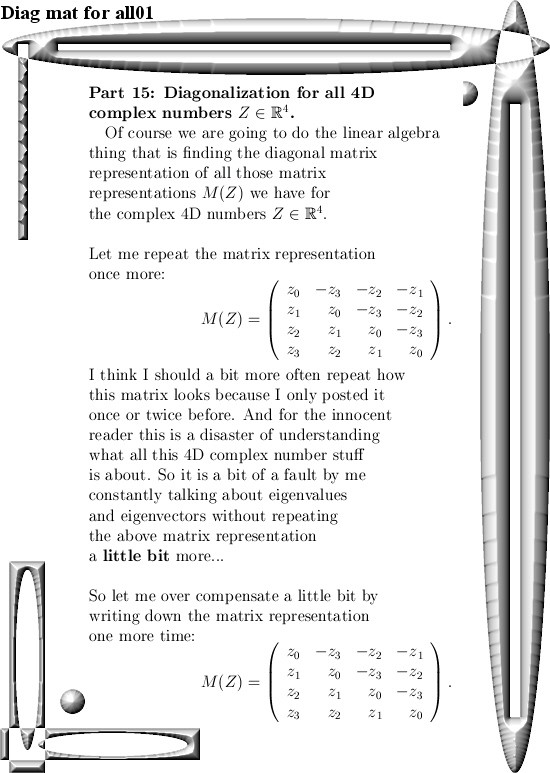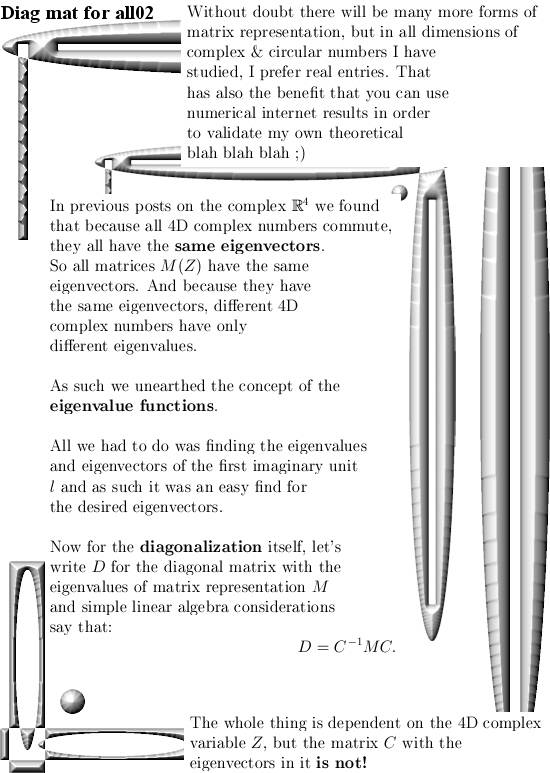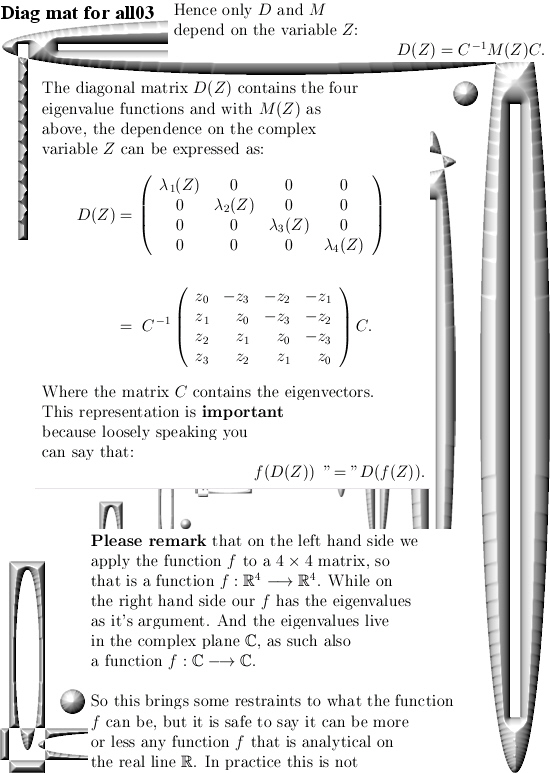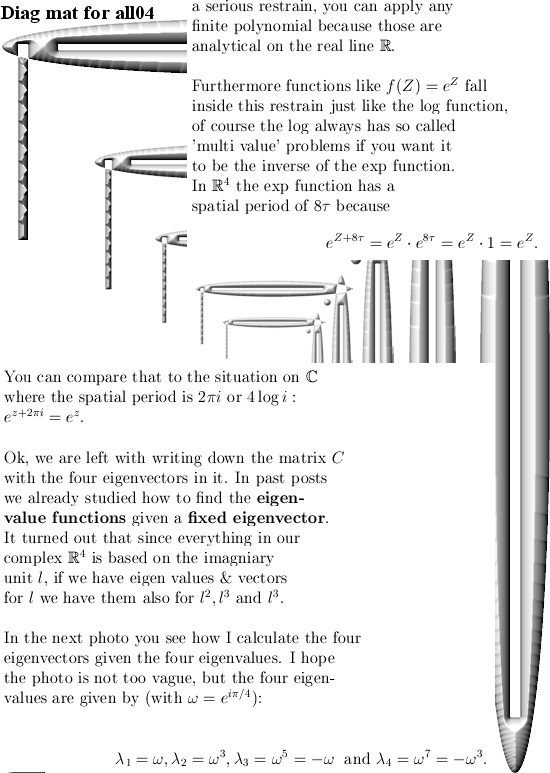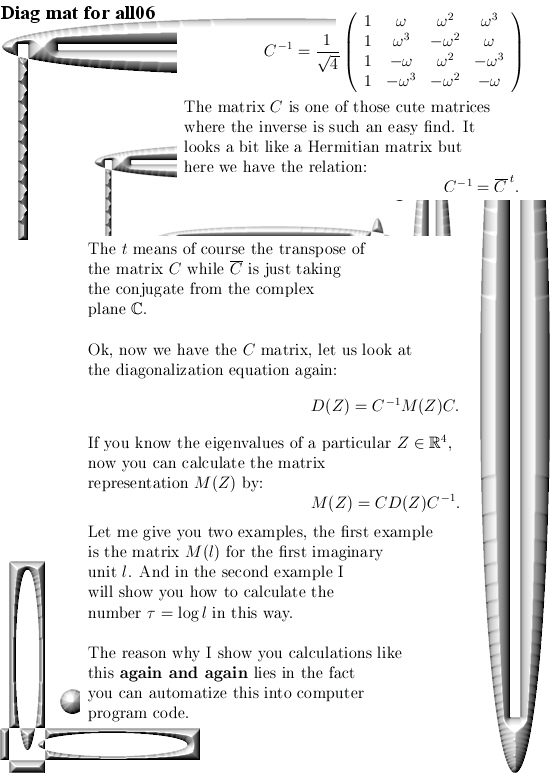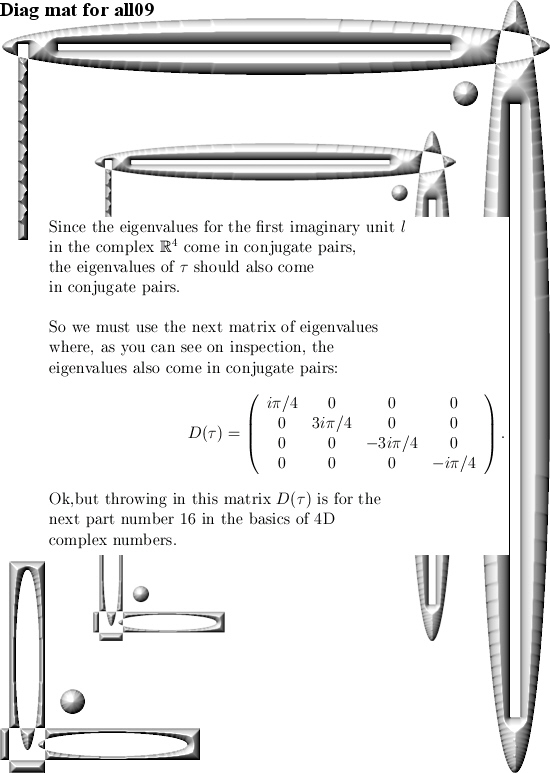That´s it, in the next post we go further with the number tau and from the eigenvalues of tau calculate the matrix representation. So see you around.

Part 14: The Cauchy integral representation for the 4D complex numbers.

It took me longer than expected to craft this update. That is also the nature of the subject; you can view and do math with Cauchy integral representation in many ways. In the end I settled on doing it just for polynomials of finite degree and even more simple: these polynomials are real valued on the real line. (So they have only real coefficients and after that are extended to the space of 4D complex numbers).

In another development, last week we had the yearly circus of Noble prizes and definitely the most cute thing ever was those evolving protein molecules. Because if you can use stuff like the e-coli bacteria you can indeed try if you can (forcefully) evolve the proteins they make… That was like WOW. Later I observed an interview with that chemistry Nobel prize winner and she stated that when she began she was told ‘gentlemen don’t do this kind of thing’.

So she neglected that ‘gentlemen stuff’ and just went on with it. That is a wise thing because if you only do what all those middle age men tell you to do you will find yourself in the very same hole as they are in…
The physics prize was also interesting, for myself speaking I was glad we did not observe those physics men totally not understanding electron spin but with the usual flair of total arrogance keep on talking about spin up and spin down.

You can also turn that spin nonsense upside down: If elementary particles only carry monopole electrical charge than why should electrons be bipolar when it comes to magnetism? That Gauss law of magnetism is only a thing for macroscopic things, there is no experimental proof it holds for quantum particles…

But let’s talk math because this update is not about what I think of electron spin. This is the second Cauchy integral representation I crafted in my life. Now the last years I produced a whole lot of math, my main file is now about 600 pages long. But only that very first Cauchy integral representation is something that I printed out on a beautiful glossy paper of size A0. That first Cauchy integral representation was on the space of 3D numbers and there life is hard: The number tau has determinant zero and as such it is not invertible. But I was able to complexify the 3D circular numbers and it was stunning to understand the number tau in that complexification of the 3D circular numbers. Just stunning…

Therefore I took so much time in trying to find an easy class of functions on the space of 4D complex numbers. I settled for easy to understand polynomials, after all any polynomial gives the same value everywhere if you write them as a Taylor series.
Since this property of polynomials is widely spread I can safely say this in this part 14 of the basics to the 4D complex numbers we have the next Theorem:
THEOREM: The math will do the talking.
PROOF: Just read the next 12 pictures. QED.

As usual all pictures are 550 x 775 pixels in size. I also use a thing I name ‘the heart of the Cauchy integral’, that is not a widely known thing so take your time so that the mathematical parts of your brain can digest it…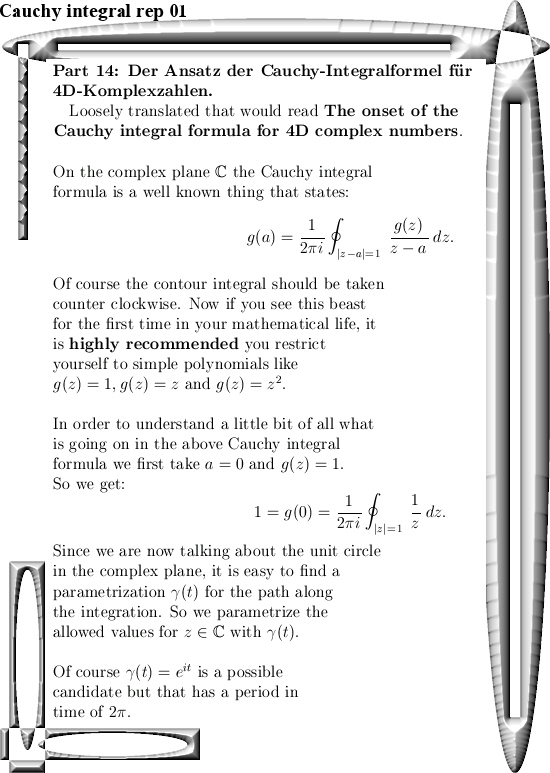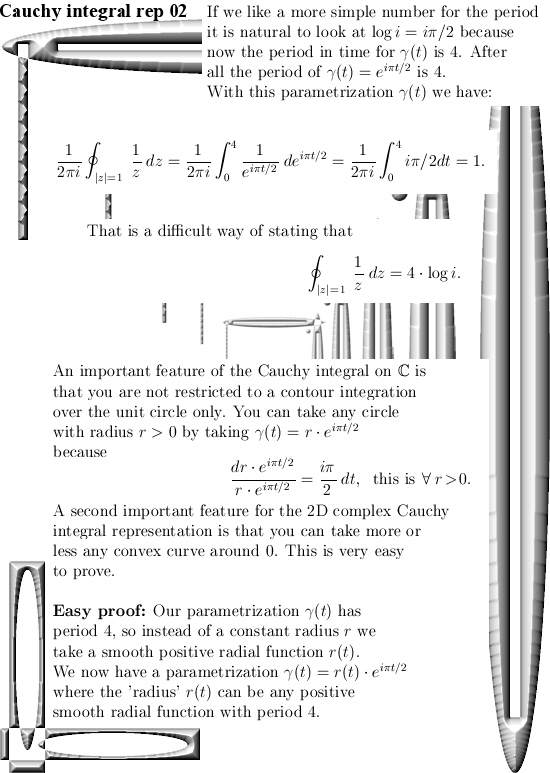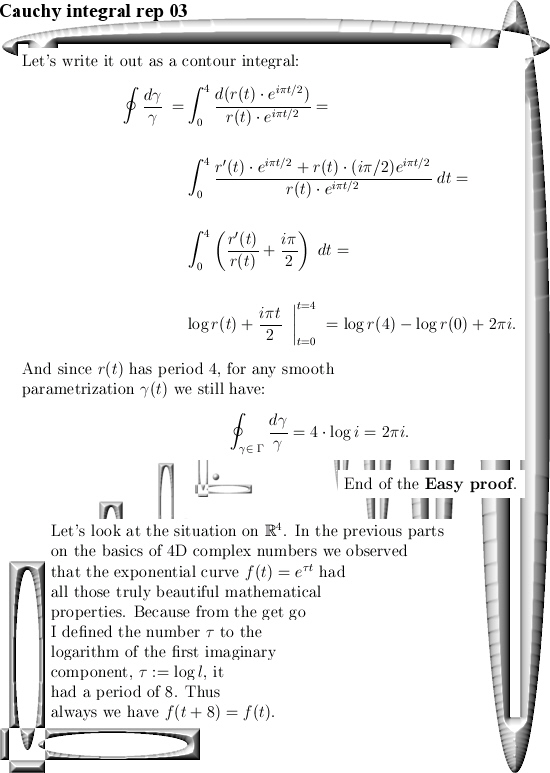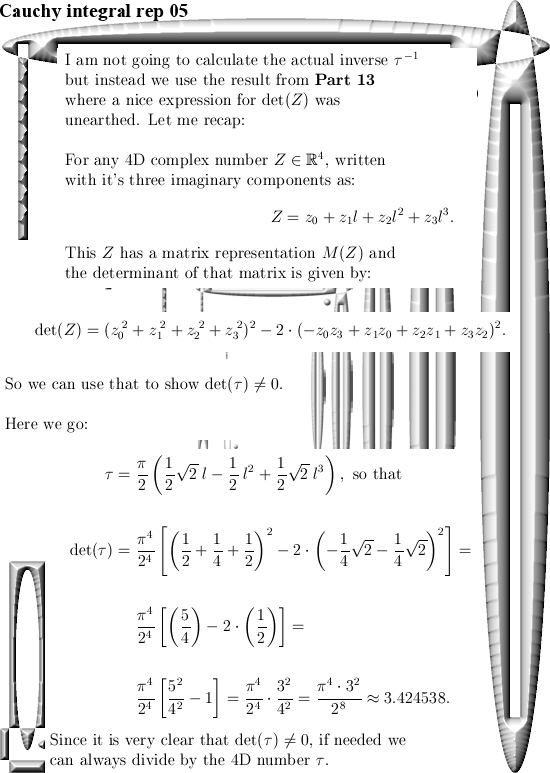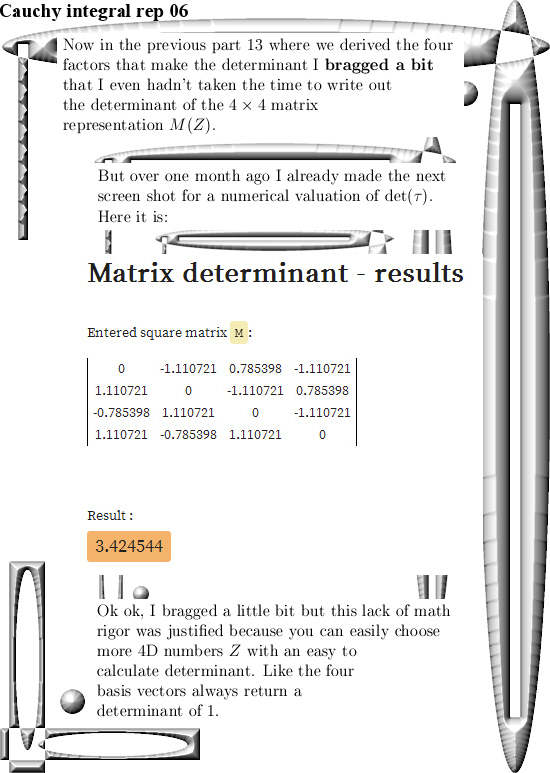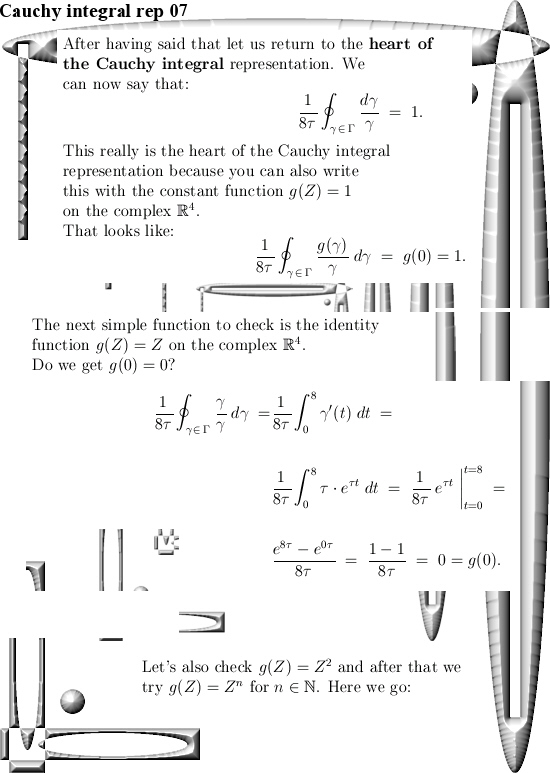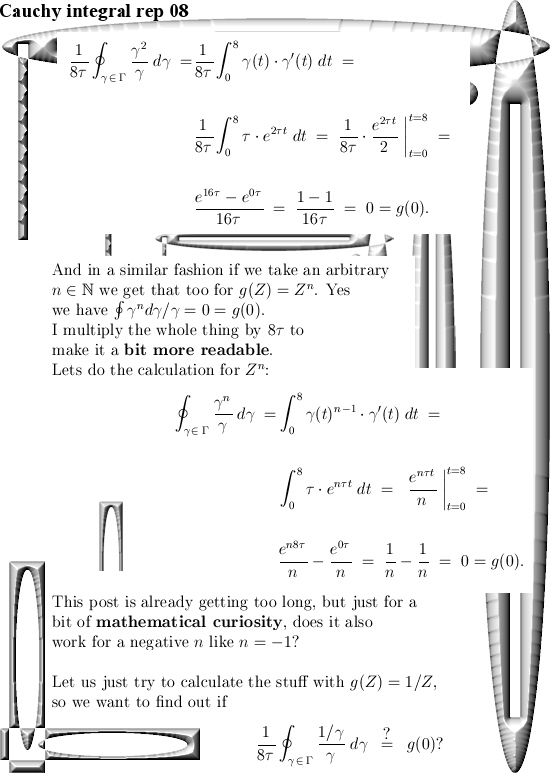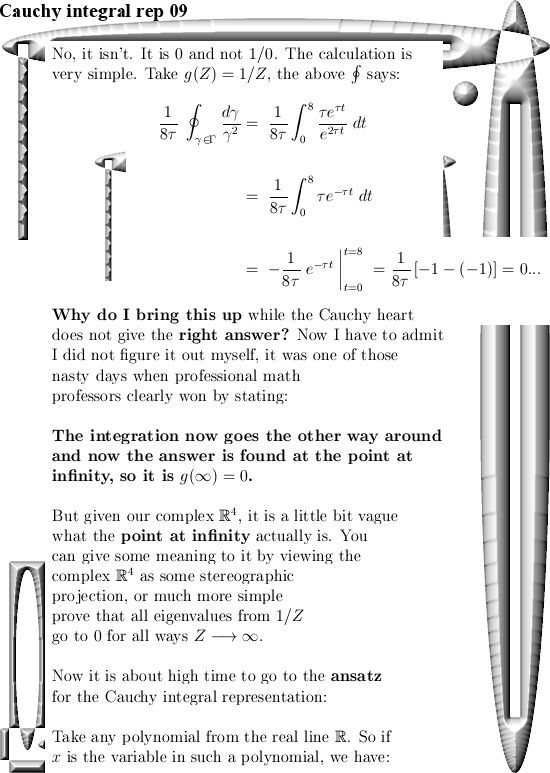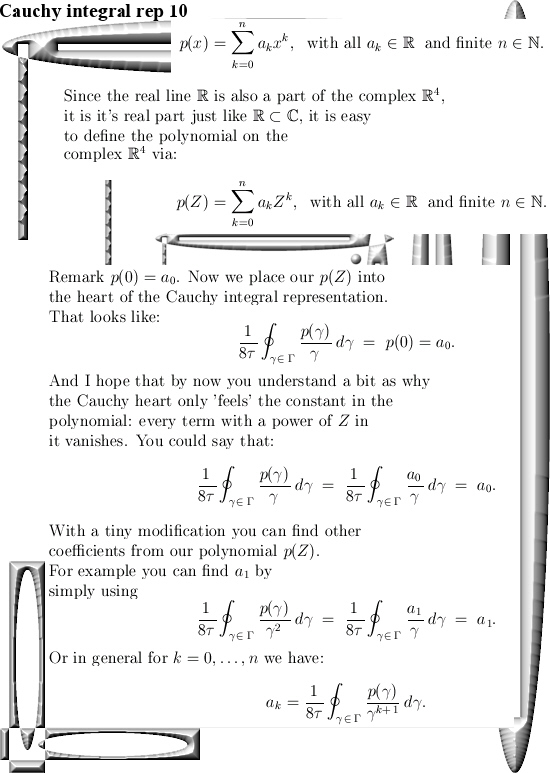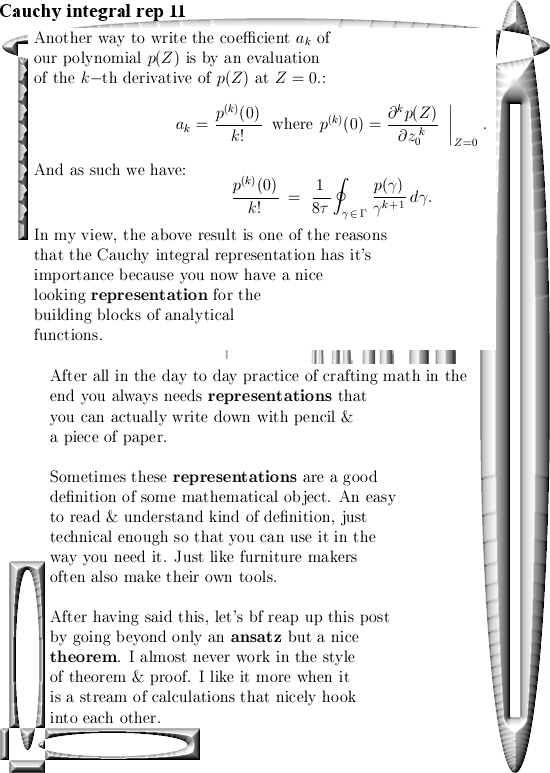I truly hope the math in this update was shallow enough so you can use it in your own path of the math that you like to explore.

End of this post, may be in Part 15 we will finally do a bit more about the diagonalization of 4D complex numbers because that is also a universal way of finding those numbers tau in the different dimensions like the 17D circular numbers & all those other spaces.

Have a nice life or try to get one.

Part 13 of the basics of 4D complex numbers: Factorization of the determinant.

It is about high time for a small update. Originally I wanted to include a little rant against all those math professors that have stated that the so called Euler formulae for the exponential circle in the complex plane is the most beautiful piece of math ever.

How can you say that and after all those other exponential circles and curves I found stay silent year in year out? We now have a fresh academic year and likely the new year nothing will happen again.

The same goes for magnetism, if it is true electrons carry magnetic charge one way or the other this will have huge economical impacts in the long run. Not only can you better understand how spintronic devices can work but as a negative also understand how nuclear fusion with those Tokomak things will never work because of the electron acceleration.

In my view this is important given the speed of climate change and how slow we react on it while at the same time we are always promised golden mountains of almost free energy if only we had nuclear fusion…

But likely university people are university people so we will just observe one more academic year of just nothing.

__________

After having said that, in this post we calculate the determinant via multiplying the four eigenvalues every 4D complex number Z has. Of course that is always when such a Z is viewed as it’s matrix representation M(Z).

You can do much more with that kind of stuff, in the previous part number 12 we unearthed the eigenvalues and eigenvectors so we can also do the nice thing of matrix diagonalization. I have not planned anything about the content in the next few parts of these small series on 4D complex numbers. So we’ll see.

This post is five pictures long, at the end I also show you the so called cylinder equation from 3D space (circular version).

Have fun reading it and thinking about it a little bit. See you around.

Before the actual post I will show you the teaser picture as published on the other website, it contains the matrix who’s determinant we are going to factor. When I read all these posts on 4D complex numbers backwards it was only in the basics number 3 where I mentioned this explicit matrix representation M(Z).

In the teaser picture you also see the main result. I never worked out the determinant of the matrix M(Z) via a method like expansion via the minors or so. Just going straightforward for the eigenvalue functions and multiply these in order to get the determinant for any 4D complex number Z.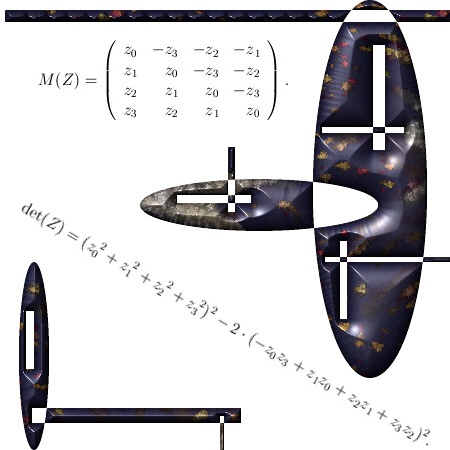So it is the determinant of the matrix representation M(Z) we are going to factorize.
Here we go: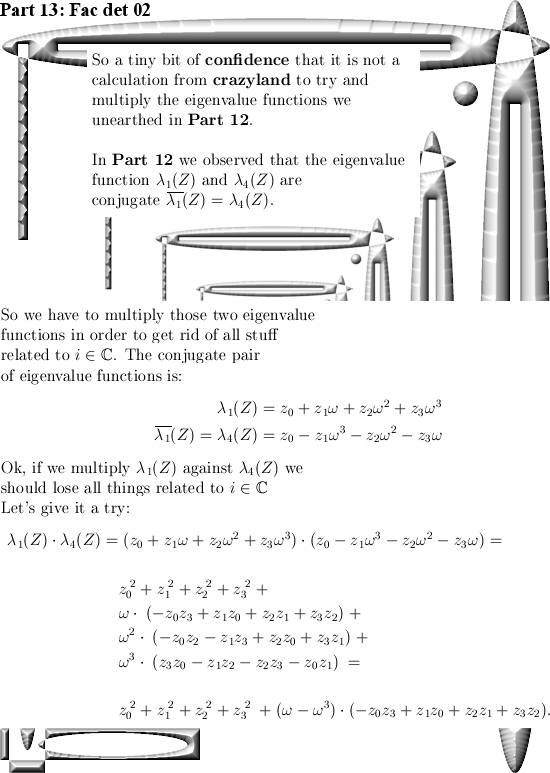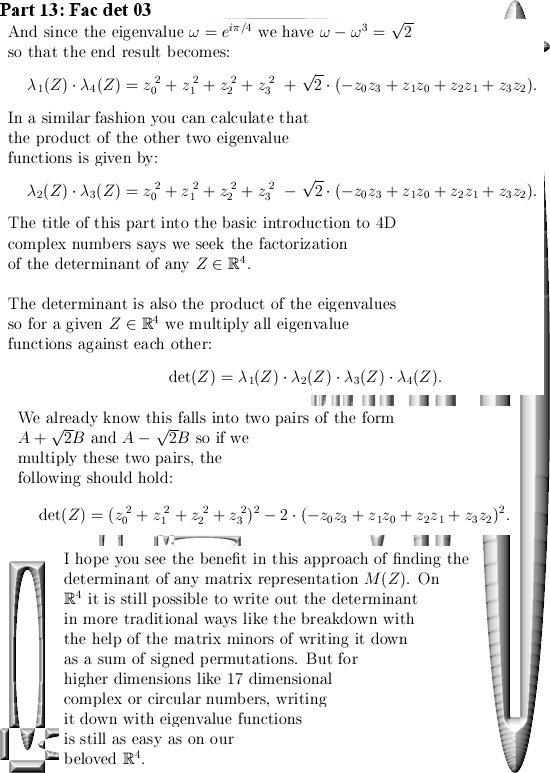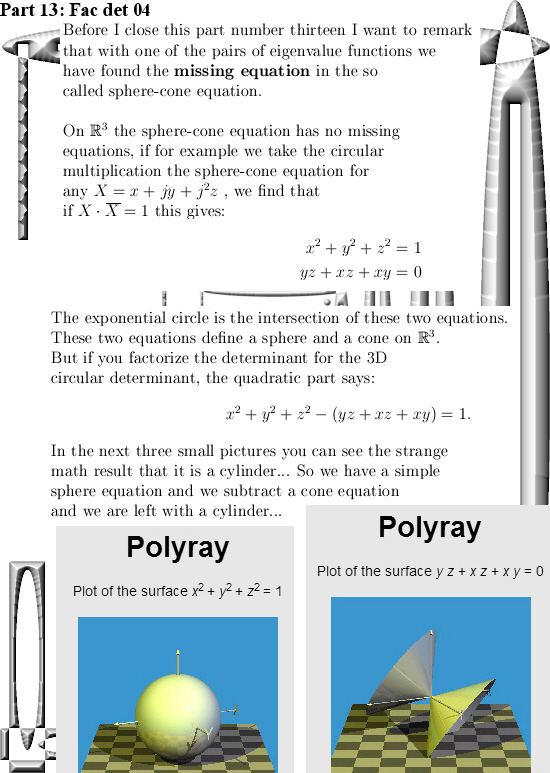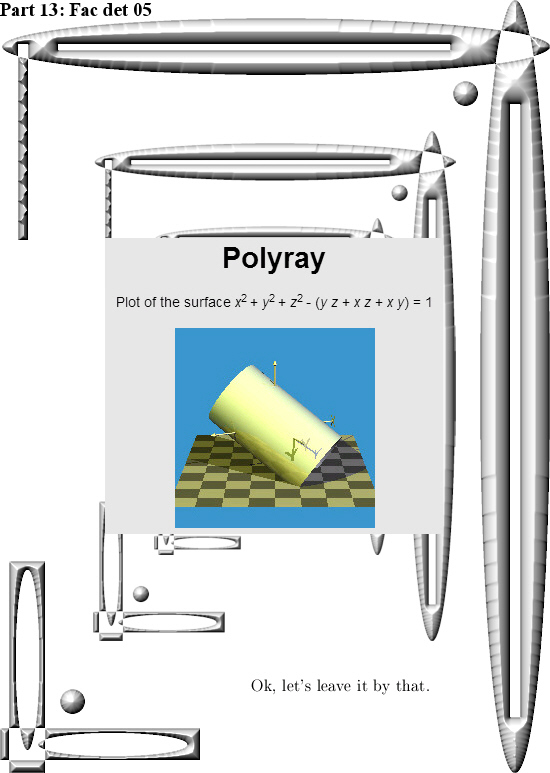Ok, let´s not rant because why waste all that emotional energy on university people?
But it is now almost your years back since I crafted a pdf about the first 10 exponential circles and curves I found:

An overview of exponential circles and curves in …
http://kinkytshirts.nl/pdfs/10_exponential_circles_and_curves.pdf

That was from 22 December 2014. We can safely conclude that at most universities a lot is happening, but it is mostly weird stuff. Weird stuff like ‘The sum of all integers equals minus 1/12…’ Oh oh, if you have people like that inside your ranks how can that bring any good?

So is it science or comedy in the next video?

It is more like comedy I just guess…

But what would life be without comedy? That would also be a strange place to live, a life without observable comedians likely is a less funny place to live in.

Hey let’s pop open one more pint of beer. By the way in the quantum world every thing is different and at first I could not believe that, why would that be? It was years and years later I found out that quantum particles like electrons never drink beer. Just never. And at that point in time I finally understood just how different the quantum world is compared to our human world.

Part 12 of the 4D complex numbers: The four eigenvalue functions for a arbitrary 4D complex number.

In Part 11 we found the eigenvalues and eigenvectors of the first imaginary unit l (with of course the property l^4 = -1). But if we have those eigenvalues for l,it is easy to find the eigenvectors of powers of l.

But every 4D complex number is a sum of a real part and the three imaginary parts with the units  l,  l ^2 and l ^3. So from a linear algebra point of view it is also easy to find the eigenvalues of such combinations of  l. Needless to say that in this post you should read  l and it powers mostly as it’s matrix representation M(l).

I have to admit that I very often avoid the constant need for the matrix representation, for example I mostly write det(l) for the determinant of M(l). But it makes texts just so unreadable if you constant write det(M(l)), I do not like that.

When you understand how to calculate the eigenvalue functions as shown below, please remark it looks a like the so called discrete Fourier transform.

This post is five pictures long (each 550×775 pixels) although the last picture is rather empty… And why no an empty picture? After all when you had a paper book in the good old days, there were always empty pages in it. Sometimes it was even written that ‘This page is left empty intentionally’. And in those long lost years that was the crime of the century because if you write on a page that it is empty, that is never true…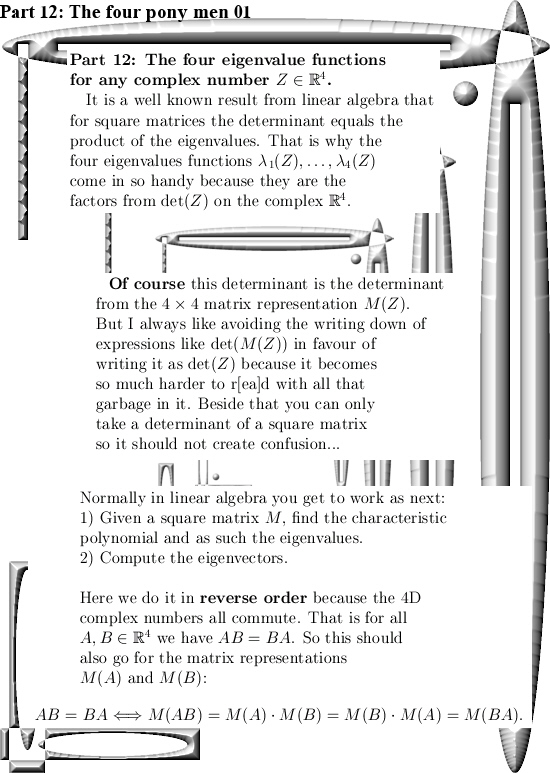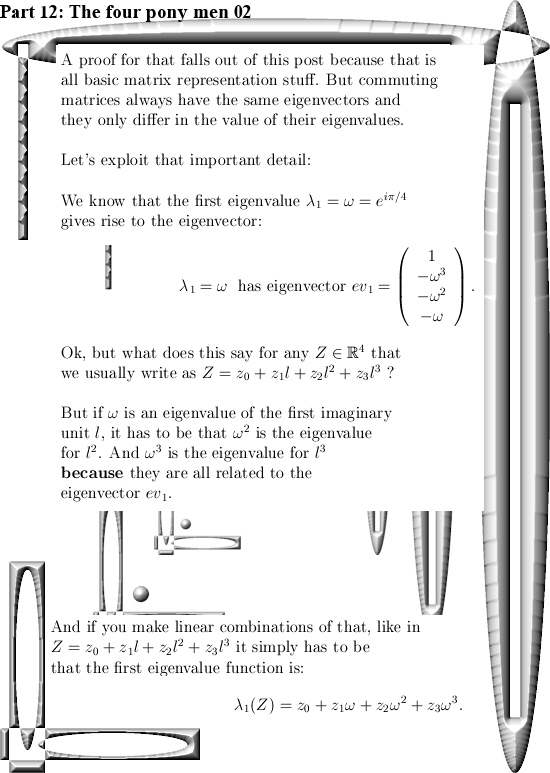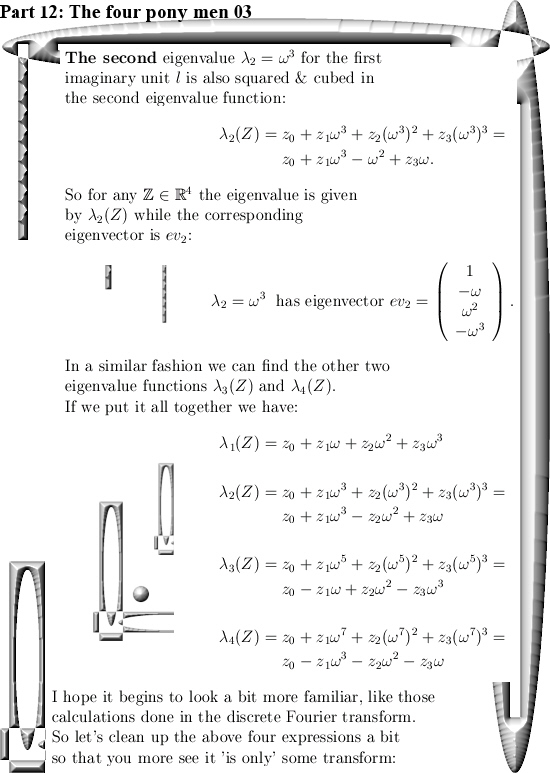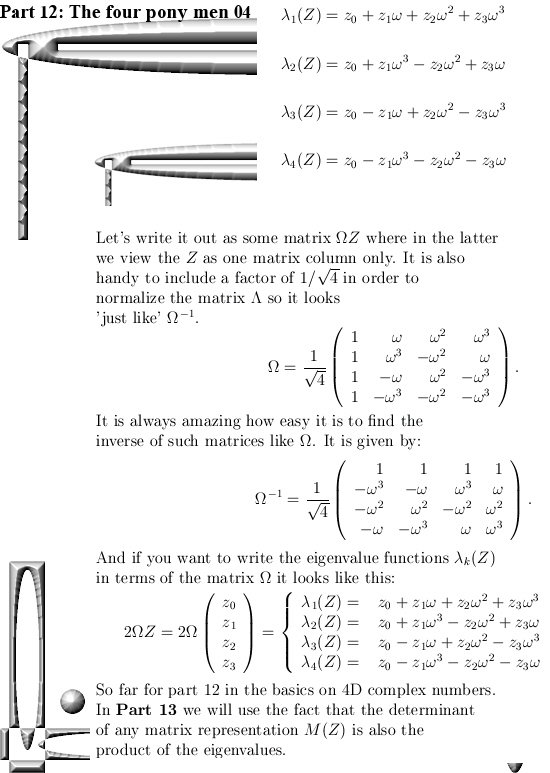In the next post we will take a look at the missing equation we still have when it comes to the calculation of the 4D exponential curve when it comes to the  sphere/cone equation. we missed one equation to arrive at a 1D solution for our exponential curve.

If you also include the demand that the determinant of the exponential curve is 1 all of the time, you can squeeze out more equations inside the 4D complex numbers.

Before we say goodbye, here is a link to the matrix representation of the discrete Fourier transform. But take you time when thinking about matrices like in the link or the Omega matrix as above.

DFT matrix
https://en.wikipedia.org/wiki/DFT_matrix

Ok, that was it. Till updates.